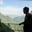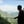Trusted answers to developer questions
Trusted Answers to Developer Questions

Related Tags

pandas
communitycreator

# What is the DataFrame.mul() method in Python?AKASH BAJWA

Grokking Modern System Design Interview for Engineers & Managers

Ace your System Design Interview and take your career to the next level. Learn to handle the design of applications like Netflix, Quora, Facebook, Uber, and many more in a 45-min interview. Learn the RESHADED framework for architecting web-scale applications by determining requirements, constraints, and assumptions before diving into a step-by-step design process.

### Overview

In Python, the DataFrame.mul() method is used to perform multiplication operations on DataFrame objects. It is an element-wise binary operation, while multiplication is denoted with an asterisk (*) operator. It also provides an additional feature to handle the missingNaNs values in one of the DataFrame objects.

### Syntax

# Signature
DataFrame.mul(other, axis = 'columns', level = None, fill_value = None)

### Parameters

It takes four parameters:

• other: This is a single or multiple element data structure or list-like object. It can be a DataFrame, series, sequence, scalar, or a constant.
• axis: This is used for deciding the axis on which the operation is applied. Whether to compare by the index (0 or index) or columns (1 or columns) that is {0 or 'index', 1 or 'columns'}.
• level: This broadcasts across a level and matches Index values on the passed multi-index level. The level could be a number or a label that marks the point at which two things have to be compared. So, it could be either an integer or a label.
• fill_value: This is used to fill missing values which are represented as NaN in the DataFrame. If we assign a number, let's say x using fill_value = x, all the missing values in the result will be filled with x.

### Return value

It returns a DataFrame obtained as a result of the arithmetic operation that is the mul() operator. In our case, we use *. As a resultwe obtain answers obtained by the multiplication of the DataFrame objects.

### Explanation

The first thing for implementation is to import pandas. Here, we import pandas as pd. So, pd will be used in place of pandas in the entire program.

### Scalar multiplication with any DataFrame

Consider we have a DataFrame object, df, containing dictionaries where country names are the keys having some values. We apply pandas DataFrame multiplication method as follows:

# importing pandas as pdimport pandas as pd# Creating a dataframe with five observationsdf= pd.DataFrame({"England":[14,4,5,4,1],				    "Pakistan":[5,2,54,3,2],				    "Australia":[20,20,7,3,8],				    "Westindies":[14,3,6,2,6]})# Print the dataframeprint(df)print()print(df.mul(2, axis = 0))
Scalar multiplication

### Explanation

• Line 4–7: We create a DataFrame containing named df having countries names as key values for dictionary.
• Line 9: We print the DataFrame.
• Line 12: We apply the mul() method as required on the DataFrame and print the result.

#### Multiplication of two DataFrames

• Line 4–7: We create df1 having countries names as key values for dictionary.
• Line 10: We print the DataFrame object, df1.
• Line 13–16: We create df2 having countries names as key values for dictionary.
• Line 19: We print the DataFrame object, df2.
• Line 23: We apply the pandas mul() method for multiplication of both DataFrame objects and print results.

Hence, we can use this method in different ways just by changing the parameters.

RELATED TAGS

pandas
communitycreator

CONTRIBUTORAKASH BAJWA

Grokking Modern System Design Interview for Engineers & Managers

Ace your System Design Interview and take your career to the next level. Learn to handle the design of applications like Netflix, Quora, Facebook, Uber, and many more in a 45-min interview. Learn the RESHADED framework for architecting web-scale applications by determining requirements, constraints, and assumptions before diving into a step-by-step design process.

Keep Exploring

Learn in-demand tech skills in half the time